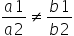Mathematics
Easy

Question

# How many solutions that the equations have when they intersect at a point?

## One solutionTwo solutionsThree solutionsInfinite solutionsHint:

## The correct answer is: One solution

### The value or values that hold true for each equation in the system constitute the solution to the system of equations. How many solutions there are for a system can be determined from the graphs of its equations.If the point of intersection is the only location where the two graphs meet when the lines cross, the coordinates of that location provide the answer to the equations involving the two variables.For example: x=y and x+2y=6This equation has only one solution as the both the lines intersects at 2,2.There are no solutions when the lines are parallel. There are always infinitely many solutions when the two equations graph as the same line.So the correct option for number of solutions that the equations have when they intersect at a point is One solution.

So here we were asked how many solutions that the equations have when they intersect at a point, so we used the concept of linear equations and understood that when two lines intersect at a point, there is only one solution.

### Related Questions to study#### With Turito Foundation.#### Get an Expert Advice From Turito.